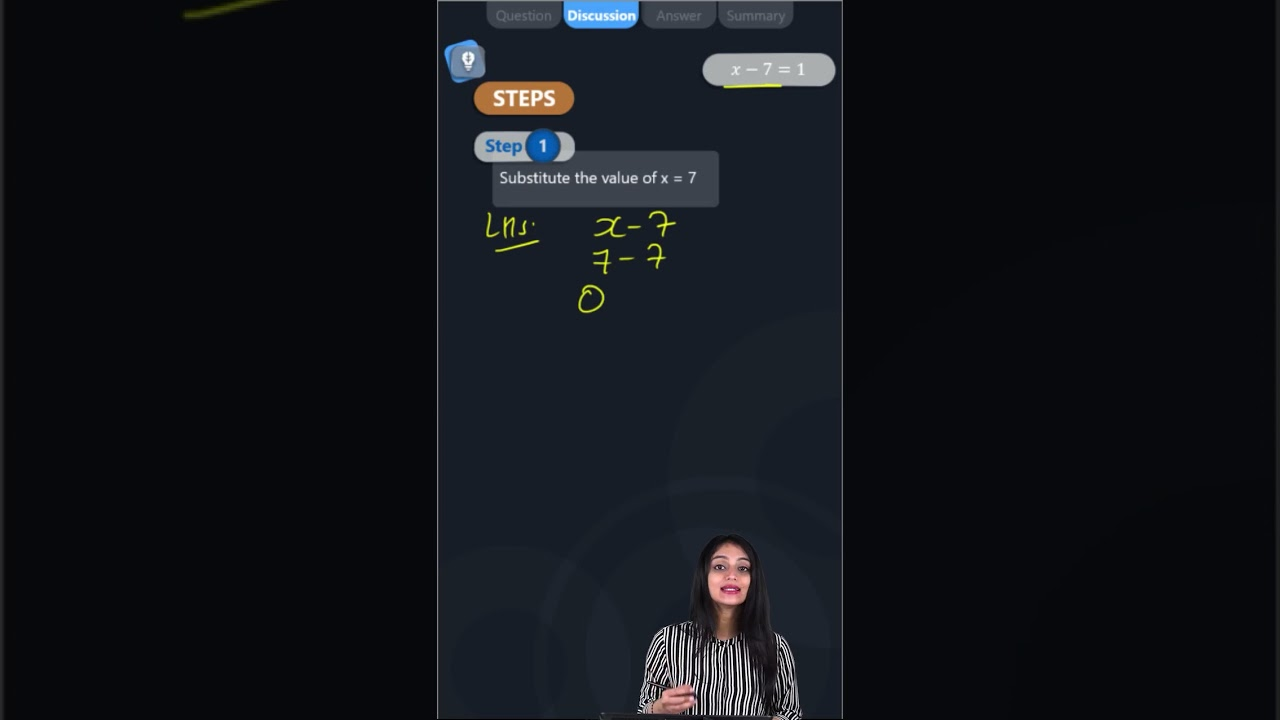Complete the last column of the table: x - 7 = 1 x = 7 Say, whether the equation is satisfied. (Yes/No)

 x – 7 = 1 x = 7 Say, whether the equation is satisfied. (Yes/No)Given: x – 7 = 1 and x = 7

Consider the LHS of the equation

LHS = x – 7

By substituting the value of x = 7

Then,

LHS = 7 – 7

LHS = 0

Consider the RHS of the given equation

RHS = 1

By comparing LHS and RHS

LHS ≠ RHS

∴ No, the equation is not satisfied.(2)(3)